Open Access ArticleThis Open Access Article is licensed under a Creative Commons Attribution-Non Commercial 3.0 Unported Licence

# Temperature mediated ‘photonic hook’ nanoparticle manipulator with pulsed illumination†

Marat Spectora, Angeleene S. Anga, Oleg V. Minin bc, Igor V. Mininbc and Alina Karabchevsky*a
aSchool of Electrical and Computer Engineering, Ben-Gurion University of the Negev, Beer-Sheva 8410501, Israel. E-mail: alinak@bgu.ac.il; maratspector@gmail.com
bNational Research Tomsk State University, Tomsk, 634050, Russia
cTomsk Polytechnic University, Tomsk, 634050, Russia

Received 5th December 2019 , Accepted 26th April 2020

First published on 27th April 2020

## Abstract

Optical forces applied on an object or cell in a non-destructive manner have revolutionised scientific instruments. Optical tweezers and atomic traps are just two representative examples. Curved forces such as photonic hooks are of particular interest for non-destructive manipulation; however, they are extremely weak in low-contrast media. Here, for the first time, we report the amplification of optical forces generated by a photonic hook via pulsed illumination mediated by temperature effects. We show that the optical force generated by the photonic hook subjected to illumination by an incident Gaussian pulse is significantly larger than the optical force generated by the photonic hook subjected to a continuous wave. We notice that under the applied photonic hook generated by a Gaussian beam, a spherical gold nanoparticle experiences a variation in its lattice temperature of ΔTl ∼ 2–4 K, leading to high index resolution. We envision that heat-associated effects can be further mitigated to achieve temperature assisted photonic hook manipulation of nanoparticles in a controllable manner by taking into account the thermo-optical properties of metals. Our findings are particularly important for tracing objects in low-contrast environments, such as optomechanically controlled drug delivery with nanoparticles in intercellular and intracellular media or cellular differentiation, to list a few examples.

## 1 Introduction

Shortly after the laser was invented, Ashkin proposed and demonstrated1 that the incident laser beam can be implemented to trap and manipulate dielectric particles. Moreover, while the traditional microscope-based trap has found applications in a wide variety of disciplines, such as biophysical research,2 this free-space optical approach is diffraction limited. Thus, achieving manipulation on the nanoscale requires auxiliary structures that generate tightly confined electric fields. Field localization has been successfully demonstrated in plasmonics research.3–7 An alternative route to sub-diffraction trapping and manipulation8–13 has been explored using high-intensity narrow light beams generated by dielectric structures, referred to as photonic nanojets.14

Photonic nanojets (PNJs) are usually generated using spherical structures subjected to illumination by a plane continuous wave (CW). When a PNJ is applied to a metallic nanoparticle, the optical forces acting on the nanoparticle result from momentum exchange.12 In addition, PNJs can also be generated using other symmetric structures.15 By breaking this symmetry, the generated structured light beams become curved. This effect is known as a photonic hook (PH)15,16 and has unique properties. Specifically, a PH experiences both lateral size and a radius of curvature as a fraction of the incident wavelength.17 PH configurations have been optimized,18 investigated for optomechanically moving nanoparticles around obstacles,16 and experimentally demonstrated.19 Previously, we explored PH-based optical manipulation under CW illumination and concluded that the generated force has a small magnitude for realistic applications.16,20 In the context of optical manipulation, CW field-based illumination causes heating of the system, which can be potentially destructive if a very large input amplitude is required.

One way to mitigate the destructive effect of CW illumination21 is by utilizing a pulsed input field.22 This largely overlooked method of increasing optical force performance has been studied numerically23 and demonstrated experimentally for femtosecond input sources for trapping latex nanoparticles.24 Pulsed illumination has also been studied for structure-based optical manipulation.25 Some applications that have been explored include the use of a pulsed laser to overcome the adhesive interaction of a particle stuck on a surface26 and as a high-speed microfluidic switch.27

Absolute trapping forces depend on the details of the beam structure and the type of illuminated wave: CW or pulsed. Here we study the photonic hook effect generated by a Gaussian pulse and its influence on the temporal dynamics of electronic and lattice temperature. Fig. 1 illustrates the studied system in which a pulsed beam, linearly polarized along the y-axis, illuminates a dielectric cuboid with broken symmetry (called a cuboid below for simplicity) with an index of n = 1.4 embedded in air. This refractive index corresponds to that of fused silica, chosen to create the optimal conditions – i.e., there needs to be a sufficient refractive index contrast between the cuboid material and the surrounding medium – for creating the photonic hook.15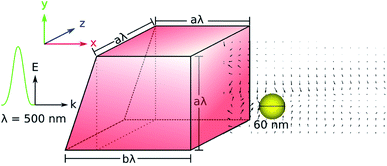Fig. 1 The dielectric cuboid, with refractive index n = 1.4, is embedded in air and irradiated by a pulsed Gaussian input field. The a/b ratio used for the cuboid is 3/4, as in ref. 16. The incident field wavelength is λ = 500 nm.

The photonic hook is generated at the output of the cuboid and is applied on a metallic probe. We utilize a gold nanoparticle (GNP) as a probe due to its biocompatibility for in vivo micro-manipulation.28 In addition, GNPs can be modified to carry cargo. To study the influence of applied pulsed illumination, we calculate the complex polarizability, α, of GNP under illumination by a Gaussian pulse as a function of temperature and search for the wavelength at which the optical force is maximal. According to ref. 29, the optical force is directly related to the imaginary part of GNP’s polarizability, α, which is shown in Fig. 2. We noticed that the imaginary part of α reaches its maximum at λ = 500 nm. This signifies the largest possible force that can be obtained with the studied particle.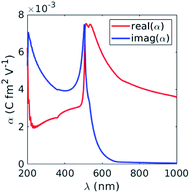Fig. 2 Real and imaginary parts of the polarizability, α (eqn (5)), of GNP subjected to a CW electric field as a function of the incident wavelength.

Since the polarizability, α, is directly linked to the particle’s scattering cross-section, σsca, by measuring the latter at different time intervals, σsca can be related to the particle displacement. In the case of temporal dynamics, the polarizability becomes time-dependent with α = α(t), and the relation to σsca(t) is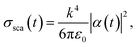(1)
where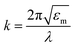is the wavevector, εm is the dielectric function of the surrounding medium, and ε0 and λ are the dielectric constant and the wavelength in free space, respectively. Thus, assuming that the initial position of the GNP is known, one can measure the scattering cross-section at different time intervals and relate it to GNP displacement. This technique can be implemented in future experiments for thermo-optomechanical manipulation and tracking metallic nanoparticles.

## 2 Results and discussion

The optical force acting on an arbitrary object is obtained by integrating the Maxwell stress tensor over a closed surface over the object.30 However, this method is computationally ineffective due to the cumbersome modelling of the object at all points where the force is obtained. To simplify the calculations, we assume that the radius of GNP, R, is much smaller than the incident wavelength, λ, i.e. Rλ, so that the particle can be approximated as an electric dipole. In this case, the force becomes
 F = (p·∇)E + ṗ × B, (2)
where p is the dipole moment and E and B are the electric and magnetic fields, respectively. The first term originates from the inhomogeneous electric field; the second term is the Lorentz force. We note that the fields in eqn (2) correspond to the exciting field – we assume that the particle represented by the dipole does not change the fields.

Taking the time average of eqn (2), we obtain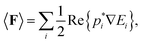(3)
and by writing the dipole moment as p = αE, we obtain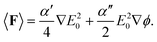(4)

The polarizability of GNP reads as α = α′ + iα′′, and ϕ reads as the phase. The first term of eqn (4) is known as the gradient force, Fgrad, responsible for trapping (or repelling) the probe particle at locations where the field intensity gradient is high. The second term is the scattering force, Fsca, which moves in parallel to the k-vector, and is responsible for unrestricted particle motion.

Eqn (4) shows that the scattering force is directly proportional to the imaginary part of the polarizability, α′′. Assuming that the probe particle is spherical, the polarizability in the Rayleigh approximation can be written as the Clausius–Mossotti relation9,31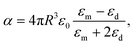(5)
where R is the radius of the probe and εm, εd, and ε0 are the dielectric constants of the surrounding medium, the probe particle, and the free space. For GNP with radius R = 30 nm, the polarizability spectrum is plotted in Fig. 2. The maximum value of α′′ is obtained at λinc = 500 nm, in the vicinity of the Au interband transition. Given that the density of Au is ρ = 19.30 g cm−3,32 we calculate the acceleration a of the nanoparticle, which is directly proportional to the force according to Newton’s second law; we plot the acceleration in Fig. 3.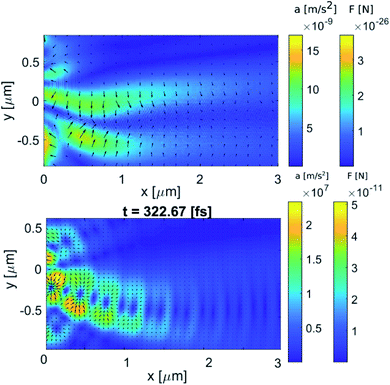Fig. 3 Calculated acceleration, a, of GNP according to eqn (6a), and forces acting on a spherical GNP with a radius of 30 nm due to (top) CW illumination and (bottom) pulsed illumination (shown here at t = 322 fs) with a wavelength of 500 nm. The optical force dynamics are shown in ESI Video 2.† The arrows indicate the direction of the optical force.

Fig. 3 shows that, contrary to CW illumination, the structure of the optical forces for pulsed radiation in the region of the photonic hook resembles a typical picture of standing waves—the presence of a chain of anti-nodes and nodes,33 located along a curved line. Specifically, the acceleration and the force for CW illumination are 15 orders of magnitude less as than for pulsed illumination. Physically, this is related to significantly larger absorbed power density by GNP, pabs,34 for the case of pulsed illumination; see eqn (8) below.

Given the acceleration in Fig. 3, we can use simple kinematics to estimate the distance traveled by the GNP during a single cycle of the electric field,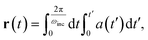(6a)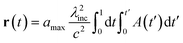(6b)
where amax is the maximum acceleration of the GNP, c is the speed of light and A(t) represents the temporal dependence of the scattered acceleration, amax. Under the CW illuminated photonic hook, the distance traveled by the GNP is diminishingly small. However, by utilizing pulsed illumination one can increase the distance traveled by the GNP.

The pulsed beam used in this work is defined by the pulsed input pre-defined in the finite difference time domain (FDTD) algorithm of Lumerical (see Methods). To better illustrate the pulse as it propagates through time and space, we set the pulse duration to 100 fs, and the time delay is arbitrarily set to 300 fs. Using the pulsed beam, we tune the incident field amplitude from 1 V m−1 to 15 MV m−1. In this case, inevitable heat effects related to the non-elastic electron–electron e–e and electron–phonon e–ph scattering must be taken into account.

To account for the interaction of the incident laser light with the metallic NP, we employ the extended Two Temperature Model (eTTM) (eqn (7)–(10)). This model describes the spatio-temporal dynamics of the non-thermal electron energy UNT, as well as of the electron and phonon (lattice) temperatures, Te and Tl, respectively. It is customary to describe the energy density evolution of NT electrons as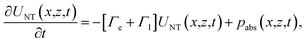(7)
where the first term on the RHS is a damping term that describes the macroscopic e–e and e–ph scattering rates represented by Γe and Γl, respectively. The second term on the RHS, pabs, describes the energy density dissipation rate. Using the Poynting theorem for dispersive media and assuming that all the photon energy is converted into heat, together with the assumption of slowly varying envelope approximation in time,34 the time-averaged absorbed power density 〈pabs〉 can be written as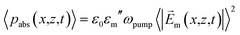(8)
where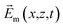is the electric field absorbed by the GNP.

For the Gaussian temporal profile of the incoming pulse, the solution of eqn (7) is

 UNT(x,z,t) = uabs(x,z,t)Z[ΓNT,τp,t], (9a)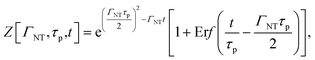(9b)
 ΓNT ≡ Γe + Γl, (9c)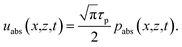(9d)
where Erf[x] is the error function.

The equations for the electron (Te) and lattice (Tl) temperatures are given as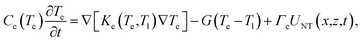(10a)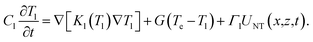(10b)
Here, C and K are the heat capacities and thermal conductivities of the electrons and the lattice, as denoted by subscripts e and l, respectively. G is the electron–phonon coupling factor related to the rate of energy exchange between the electrons and the lattice. We note that the phonon thermal conductivity Kph(Tl) is much smaller than the electron thermal conductivity Kel(Te,Tl); henceforth, it is neglected.

We now solve eqn (7)–(10) numerically with the parameters suitable for Au specified in Table 1.

Table 1 Parameters used in the numerical solution of the eTTM (eqn (10))
Parameter Value Units Reference
C eqe 2.1 × 104 J m−3 K−1 35
C l 2.5 × 106 J m−3 K−1 35
G 2.5 × 1016 J m−3 K−1 s−1 36
Γ e 2 × 1012 s−1 37
Γ l 1 × 1012 s−1 37
Γ NT 3 × 1012 s−1 37
Γ T l 1 × 1010 s−1 37
Γ T e 1.2 × 1012 s−1 37
λ pump 500 nm
τ pump 100 fs
n d 1

Assuming that the variation of the electron (Te) and the lattice (Tl) temperatures is small with respect to their initial value (Te = Tl = Tin = 300 K), we compare the numerical solution of eqn (7)–(10) to the analytical one given by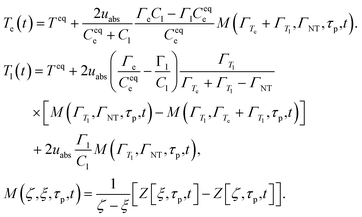(11)
where uabs represents the energy density absorbed by the GNP. It is given by the following expression: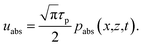(12)

The calculated electronic (Te) and lattice (Tl) temperature dynamics are shown in Fig. 4. We note that Fig. 4 shows the calculated results for Te and Tl when the GNP is subject to uniform Gaussian pulse excitation. The duration of the pulse is τp = 0.1 ps, the wavelength of excitation is λ = 500 nm, and the absorbed electric field is Em = 15 MV m−1.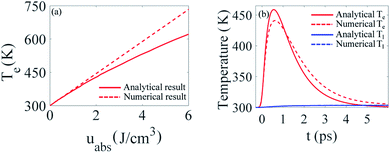Fig. 4 (a) Maximum temperature of electrons Te as a function of absorbed energy density. (b) Temporal dynamics of electronic (Te) and lattice (Tl) temperatures due to the uniform excitation of spherical GNP by a Gaussian pulse at λ = 500 nm. The duration of the pulse is τp = 0.1 ps and the absorbed electric field is Em = 15 MV m−1.

We see that both the analytical and the numerical results for the maximum values of Te show a linear dependence on uabs for low energy densities. In this case, the deviation between the analytical and the numerical result is less than 5%. However, further increasing uabs leads to higher values of Te, which in turn limits the validity of our assumption, namely, that the variation of the electron temperature, Te, is small with respect to its initial value (Te = Tin = 300 K). This in turn leads to a deviation of 25% between the analytical and the numerical results.

To account for the temporal dynamics of the dielectric function of GNP, εm(Te,Tl), we use the temperature-dependent permittivity model described in detail in ref. 38. Specifically, the GNP is excited at λ = 500 nm, which is in the vicinity of the interband transition of Au.39 Thus, we take into account the interband (εinter(Te,Tl)) and intraband (εintra(Te,Tl)) contributions to the dielectric function of Au as a function of time, which is represented in Fig. 5.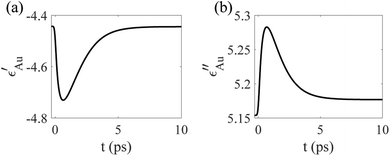Fig. 5 Temporal dynamics of the (a) real and (b) imaginary part of ε as a result of uniform excitation at λ = 500 nm. The duration of the pump pulse is τp = 0.1 ps and the absorbed electric field is Em = 15 MV m−1.

Furthermore, by taking into account the temporal dynamics of the interband and intraband dielectric functions, the temporal dependence of the particle polarizability, α, is easily obtained from eqn (5), and is shown in Fig. 6. According to eqn (4), the optical force depends on the polarizability of the GNP, which becomes diminishingly small after 10 ps. Thus, in the temporal range of interest, the thermal dissipation effect of the metallic particle need not be considered, since the relaxation of the lattice temperature occurs on a much slower time scale.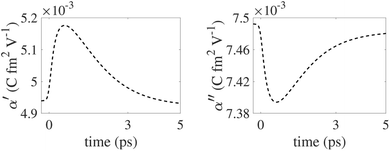Fig. 6 Dynamics of α′ (left) and α′′ (right) as a result of uniform excitation at λ = 500 nm (eqn (5)). The duration of the pump pulse is τp = 0.1 ps and the absorbed electric field is Em = 15 MV m−1.

As it interacts with the dielectric cuboid, the pulsed field generating the photonic hook is shown in ESI Video 1. From the electric and magnetic fields generated by the simulation, we then use eqn (2) to calculate the force, along with the time-dependent polarizability shown in Fig. 6. The optical force acting on the probe GNP is shown in ESI Video 2. Integrating the acceleration with respect to time, the velocity of the GNP after the pulsed field excitation dissipates at t ≈ 500 fs is shown in Fig. 7.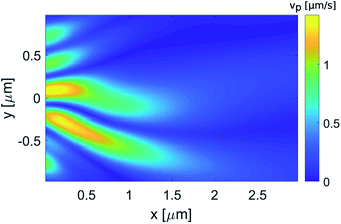Fig. 7 Calculated velocity, vpar, of GNP subject to the pulsed illumination. The incident wavelength is λ = 500 nm and the radius of the GNP is R = 30 nm.

## 3 Purcell factor calculation

The ability to track particles in inter- and intracellular media is of the highest importance for revealing molecular transport, studying fundamental biological mechanisms and drug delivery, to list a few examples. However, the main limitation of cellular medium is its optical low contrast. To track the GNP studied here in low-contrast medium, we propose first encapsulating it with a photoluminescent emitter.40,41 Once encapsulated with an emitter, a possible implementation can be accomplished by measuring the dynamically varying Purcell factor. The Purcell factor, Fp,42 can be defined as the ratio between the density of surface plasmon modes, ρSP, and the density of modes in free space, ρfree,43 namely,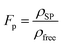(13)

The Purcell factor depends on the dielectric function of the GNP, εm (which in our case is time-dependent), and of the surrounding media, εd. By taking into account the temporal dynamics of the dielectric function of Au and utilizing the approach of Sun et al.,43 we calculate the wavelength dependence of the Purcell factor, Fp, at different time periods Δt after excitation by a pulsed laser beam (Fig. 8). We found that the spectral location of the maximum value of the Purcell factor is shifted by 6 nm due to the heating effect of the ‘hot’ GNP as compared to the ‘cold’ one. This important observation can be utilized for tracking the GNP when it is in intercellular medium (n = 1.33) and when it passes the cellular phospholipid membrane (n = 1.34).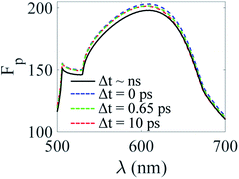Fig. 8 Purcell factor as a function of incident wavelength at different time intervals Δt after illumination by a Gaussian pulse with incident wavelength λ = 500 nm. The duration of the pump pulse is τp = 0.1 ps and the absorbed electric field is Em = 15 MV m−1. The particle is embedded in a medium with a refractive index of n = 1.33.

## 4 Methods

The electric and magnetic fields generated by the plane wave incident on the cuboid are simulated using Lumerical FDTD. We used the scattered field formalism with the source offset by an arbitrarily selected amount (300 fs) in order to input the full Gaussian signal into the system. The simulation is bounded by perfectly matched layers on all sides. We used a mesh size of 5 nm along both x and y in the shadow region (the mesh along z was left as default) and a monitor with a time sampling rate of 5728.89 THz (corresponding to a minimum sampling rate per cycle of 10), in order to obtain a reasonably-sized dataset of electric and magnetic field data. The resulting dataset was then input in Matlab, where the field derivatives were obtained using the central difference method.

## 5 Conclusion

To conclude, we reported on an amplification of optical forces generated by a photonic hook applied on a spherical nanoparticle via pulsed illumination mediated by temperature effects. We analyzed the influence of a Gaussian pulse applied on a spherical GNP. Importantly, the magnitude of the optical force acting on the gold nanoparticle and the velocity achieved by the GNP subject to the pulsed illumination are greater than the force and velocity of the same GNP under CW illumination. We proposed a novel tracking concept for particle movement in low-contrast media. We noticed that the effective scattering cross-section of the heated particle depends on the intensity of the input laser beam; therefore the scattering cross-section of the particle can be tuned for its tracking. In addition, as a possible implementation of tracking a nanoparticle in low index contrast medium, we analyzed the wavelength dependence of the Purcell factor, Fp, at different time intervals after excitation by a Gaussian pulse. We found that by illuminating the GNP with a Gaussian pulse at 500 nm and increasing the refractive index of the GNP surrounding media by 0.75%, the Purcell factor (Fp) peak experiences a spectral shift of 6 nm. An experimental analogue of the Purcell factor, Fp, is the measurement of the recombination rate enhancement, which is defined as the ratio between the total recombination rate of the photoluminescent emitter encapsulated GNP and the total recombination rate of the bare photoluminescent emitter.44 The reported photonic hook can easily be extended to other types of localized structure beams.

## Conflicts of interest

There are no conflicts of interest to declare.

## Acknowledgements

This work was partially funded by the Israel Innovation Authority KAMIN program grant number 69073. The work was partially carried out within the framework of the Tomsk Polytechnic University Competitiveness Enhancement Program, Russia, and was partially supported by the RFBR Grant No. 20-57-S52001.

## Notes and references

1. A. Ashkin, Phys. Rev. Lett., 1970, 24, 156–159 CrossRef CAS.
2. J. E. Baker, R. P. Badman and M. D. Wang, Wiley Interdiscip. Rev.: Nanomed. Nanobiotechnol., 2018, 10, e1477 Search PubMed.
3. T. Shoji and Y. Tsuboi, J. Phys. Chem. Lett., 2014, 5, 2957–2967 CrossRef CAS PubMed.
4. W. Zhang, L. Huang, C. Santschi and O. J. F. Martin, Nano Lett., 2010, 10, 1006–1011 CrossRef CAS PubMed.
5. V. Pacheco-Peña, I. V. Minin, O. V. Minin and M. Beruete, Ann. Phys., 2016, 528, 684–692 CrossRef.
6. A. Ivinskaya, M. I. Petrov, A. A. Bogdanov, I. Shishkin, P. Ginzburg and A. S. Shalin, Light: Sci. Appl., 2017, 6, e16258 CrossRef CAS PubMed.
7. I. V. Minin, O. V. Minin, D. S. Ponomarev and I. A. Glinskiy, Ann. Phys., 2018, 530, 1800359 CrossRef.
8. A. Kovrov, A. Novitsky, A. Karabchevsky and A. S. Shalin, Ann. Phys., 2018, 530, 1800129 CrossRef.
9. I. V. Minin, O. V. Minin, Y. Cao, Z. Liu, Y. E. Geints and A. Karabchevsky, Sci. Rep., 2019, 9, 12748 CrossRef PubMed.
10. Y.-C. Li, H.-B. Xin, H.-X. Lei, L.-L. Liu, Y.-Z. Li, Y. Zhang and B.-J. Li, Light: Sci. Appl., 2016, 5, e16176 CrossRef CAS PubMed.
11. H. Wang, X. Wu and D. Shen, Opt. Lett., 2016, 41, 1652–1655 CrossRef PubMed.
12. X. Cui, D. Erni and C. Hafner, Opt. Express, 2008, 16, 13560–13568 CrossRef CAS PubMed.
13. M. Dienerowitz, M. Mazilu and K. Dholakia, J. Nanophotonics, 2008, 2, 021875 CrossRef.
14. B. S. Luk’yanchuk, R. Paniagua-Domínguez, I. V. Minin, O. V. Minin and Z. Wang, Opt. Mater. Express, 2017, 7, 1820 CrossRef.
15. I. V. Minin and O. V. Minin, Diffractive Optics and Nanophotonics, Springer International Publishing, Cham, 2016 Search PubMed.
16. A. S. Ang, A. Karabchevsky, I. V. Minin, O. V. Minin, S. V. Sukhov and A. S. Shalin, Sci. Rep., 2018, 8, 2029 CrossRef PubMed.
17. K. Dhloakia and G. D. Bruce, Nat. Photonics, 2019, 13, 229–230 CrossRef.
18. L. Yue, O. V. Minin, Z. Wang, J. N. Monks, A. S. Shalin and I. V. Minin, Opt. Lett., 2018, 43, 771–774 CrossRef PubMed.
19. I. V. Minin, O. V. Minin, G. M. Katyba, N. V. Chernomyrdin, V. N. Kurlov, K. I. Zaytsev, L. Yue, Z. Wang and D. N. Christodoulides, Appl. Phys. Lett., 2019, 114, 031105 CrossRef.
20. A. S. Ang, I. V. Minin, O. V. Minin, S. V. Sukhov, A. S. Shalin and A. Karabchevsky, Proc. of the 9th Int. conf. on Metamaterials, Photonic crystals and Plasmonics, 2018 Search PubMed.
21. K. Setoura, Y. Okada and S. S. Hashimoto, Phys. Chem. Chem. Phys., 2014, 16, 26938–26945 RSC.
22. C. Schaffer, A. Brodeur and E. Mazur, Meas. Sci. Technol., 2001, 12, 1784–1794 CrossRef CAS.
23. N. du Preez-Wilkinson, A. B. Stilgoe, T. Alzaidi, H. Rubinsztein-Dunlop and T. A. Nieminen, Opt. Express, 2015, 23, 7190–7208 CrossRef CAS PubMed.
24. A. K. De, D. Roy, A. Dutta and D. Goswami, Appl. Opt., 2009, 48, G33–G37 CrossRef PubMed.
25. B. J. Roxworthy and K. C. J. Toussaint, Sci. Rep., 2012, 2, 660 CrossRef PubMed.
26. J.-l. Deng, Q. Wei, Y.-z. Wang and Y.-q. Li, Opt. Express, 2005, 13, 3673–3680 CrossRef PubMed.
27. T.-H. Wu, L. Gao, Y. Chen, K. Wei and P.-Y. Chiou, Appl. Phys. Lett., 2008, 93, 144102 CrossRef.
28. F. Hajizadeh and S. Reihani, Opt. Express, 2010, 18, 551–559 CrossRef CAS PubMed.
29. K. Svoboda and S. M. Block, Annu. Rev. Biophys. Biomol. Struct., 1994, 23, 247–285 CrossRef CAS PubMed.
30. L. Novotny and B. Hecht, Principles Of Nano Optics, 2006 Search PubMed.
31. K. Svoboda and S. M. Block, Opt. Lett., 1994, 19, 930–934 CrossRef CAS PubMed.
32. J. Steehler, J. Chem. Educ., 1997, 74, 378 CrossRef.
33. I. Minin, O. Minin, V. Pacheco-Peña and M. Beruete, Quantum Electron., 2016, 46, 555–557 CrossRef CAS.
34. J. D. Jackson, Classical electrodynamics, Wiley & Sons, 3rd edn, 1998 Search PubMed.
35. N. W. Ashcroft and N. D. Mermin, Solid State Physics, Saunders College, Philadelphia, 1976 Search PubMed.
36. P. Allen, Phys. Rev. Lett., 1987, 59, 1460–1463 CrossRef CAS PubMed.
37. C. Sun, F. Vallee, L. Acioli, E. Ippen and J. G. Fujimoto, Phys. Rev. B: Condens. Matter Mater. Phys., 1993, 48, 12365–12368 CrossRef CAS PubMed.
38. T. Stoll, P. Maioli, A. Crutt, G. Wurtz and A. Zayatz, Nano Lett., 2012, 12, 1561–1565 CrossRef PubMed.
39. M. Guerrisi, R. Rosei and P. Winsemius, Phys. Rev. B: Solid State, 1975, 12, 557–563 CrossRef CAS.
40. A. Karabchevsky, S. Karabchevsky and I. Abdulhalim II, J. Nanophotonics, 2011, 5, 051813 CrossRef.
41. I. Abdulhalim, A. Karabchevsky, C. Patzig, B. Rauschenbach, B. Fuhrmann, E. E. Eltzov, R. Marks, J. J. Xu, F. Zhang and A. Lakhtakia, Appl. Phys. Lett., 2009, 94, 063106 CrossRef.
42. E. M. Purcell, in Spontaneous Emission Probabilities at Radio Frequencies, Springer US, Boston, MA, 1995, pp. 839–839 Search PubMed.
43. G. Sun, J. B. Khurgin and R. A. Soref, J. Opt. Soc. Am. B, 2008, 25, 1748–1755 CrossRef CAS.
44. I. Gontijo, M. Boroditsky, E. Yablonovitch, S. Keller, U. K. Mishra and S. P. DenBaars, Phys. Rev. B: Condens. Matter Mater. Phys., 1999, 60, 11564 CrossRef CAS.

### Footnotes

Electronic supplementary information (ESI) available: (1) acceleration.avi and (2) force.avi. See DOI: 10.1039/c9na00759h
These authors contributed equally to the work.
 This journal is © The Royal Society of Chemistry 2020